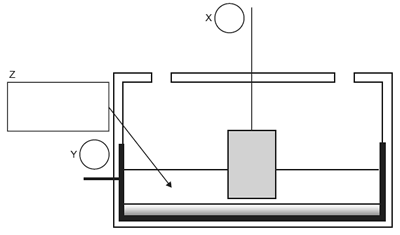Electrolysis 2006 VCE Tin metal is electroplated onto sheets of iron using a tin anode and a well-stirred solution containing Sn2+ ions. During this process A. the anode increases in mass. B. Sn2+ ions move towards the cathode. C. the concentration of Sn2+ in the solution decreases. D. the concentration of Sn2+ in the solution increases. Solution The passage of 0.019 faradays of electricity through a molten chromium compound yields 0.50 g of chromium metal. The oxidation number of chromium in the compound is likely to be A. +2 B. +3 C. +4 D. +6 Solution The simplified diagram below shows the Hall Cell that is used for the industrial production of aluminium.In circles X and Y in the diagram, show the polarity of the electrodes. Solution In the Hall Cell, the electrolyte consists of alumina mixed with another compound. In box Z, write the name or the formula of this compound. Solution Write a half equation for the reaction that occurs at the i. anode Solution ii. cathode. Solution Suppose the electrolyte was replaced with an aqueous solution of Al(NO3)3 at 25°C. Write an equation for the half reaction that would occur at the cathode. SolutionGive concise explanations in answer to each of the following. a. Calcium chloride is added to the molten sodium chloride electrolyte in the Downs Cell for the production of sodium. Explain why calcium metal is not produced in the cell. b. An asbestos barrier once used in commercial diaphragm cells to separate the chemicals produced by the electrolysis of concentrated salt water is replaced with a plastic membrane in modern cells. Give one advantage of using a plastic membrane instead of asbestos. c. The heat of combustion of plant-based foodstuffs as determined by calorimetry is very often an overestimate of the energy available when the food is eaten and digested by humans. Give one reason why this might be the case. Solution